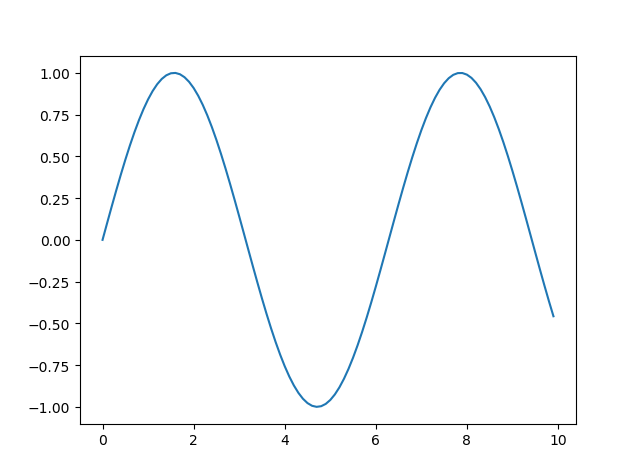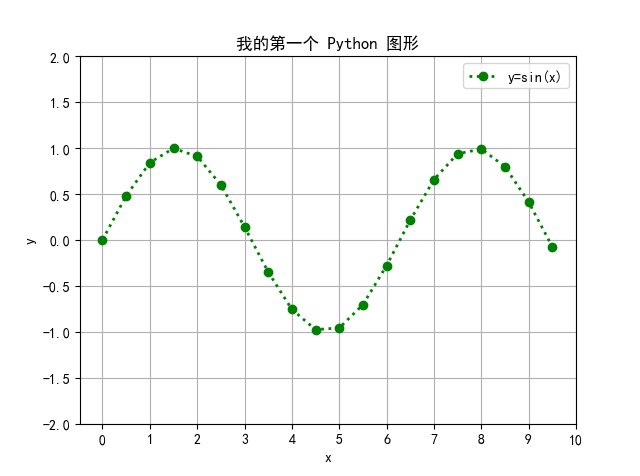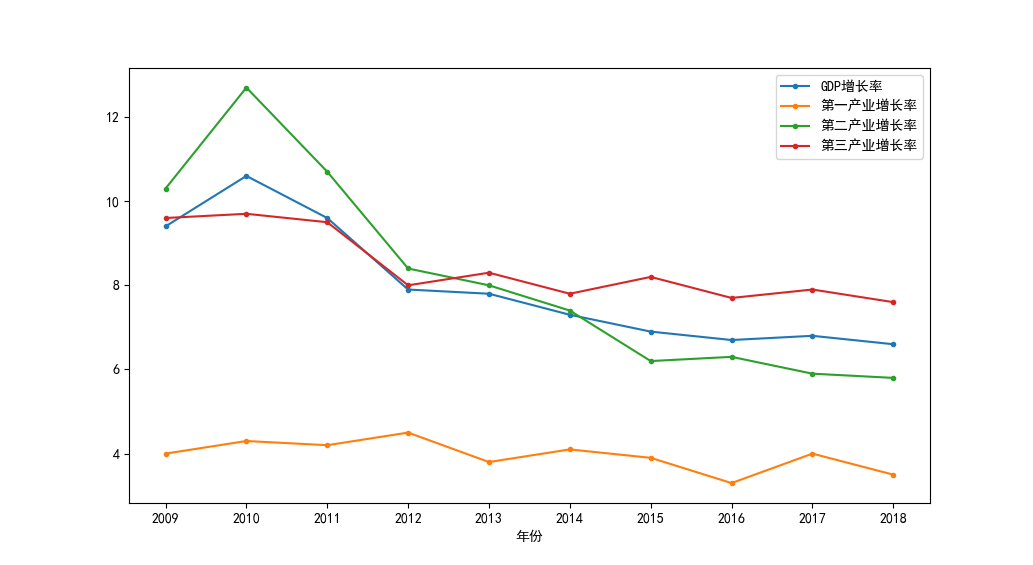• 1 在文件1里面使用plot画图时，如果import了文件2，并且文件2里面也用到Plot画图。这时，就算在文件1里面设置了Plot的style，该设置的格式仍然会在文件2里面被改变。
1
在文件1里面使用plot画图时，如果import了文件2，并且文件2里面也用到Plot画图。这时，就算在文件1里面设置了Plot的style，该设置的格式仍然会在文件2里面被改变。
展开全文• 今天小编就为大家分享一篇解决python中使用plot画图,图不显示的问题，具有很好的参考价值，希望对大家有所帮助。一起跟随小编过来看看吧
• 文章目录1....使用 matplotlib 包画图时，我们一般加载里面的 pyplot，并命名为 plt，然后使用 plot 函数画图。 # 导入 matplotlib 中的 plot， 并命名为常用名 plt import matplotlib.pyplot as p...


文章目录
1. 加载宏包2. plot 函数的语法plot([x], y, [fmt], **kwargs)

3. 一张图画好几条线

1. 加载宏包
使用 matplotlib 包画图时，我们一般加载里面的 pyplot，并命名为 plt，然后使用 plot 函数画图。
# 导入 matplotlib 中的 plot， 并命名为常用名 plt
import matplotlib.pyplot as plt

例如，下面的代码画出正弦函数

y

=

s

i

n

(

x

)

y=sin(x)

的图形。
import matplotlib.pyplot as plt
import numpy as np

# 生成数据
x = np.arange(0, 10, 0.1) # 横坐标数据为从0到10之间，步长为0.1的等差数组
y = np.sin(x) # 纵坐标数据为 x 对应的 sin(x) 值

# 生成图形
plt.plot(x, y)

# 显示图形
plt.show()

图形显示：利用plot函数，我们可以对图形进行更多精细的设置，官方的详细文档可以参看：https://matplotlib.org/3.1.1/api/_as_gen/matplotlib.pyplot.plot.html。
2. plot 函数的语法
Plot 函数的基本语法是：

plot([x], y, [fmt], **kwargs)

含义[x]可选参数，横坐标轴数据y纵坐标轴数据[fmt]可选参数，字符串，定义图形的基本样式：颜色，点形，线形**Kwargs不定长的关键字参数，用字典形式设置图形的其他属性
[fmt] 的常用代码（包括颜色代码、点形代码、线形代码），由下面的表所示。 颜色：
代码颜色‘b’蓝色‘g’绿色‘r’红色‘y’黄色‘k’黑色‘w’白色
点形：
代码点形‘.’圆点形‘o’实心圆‘*’星号‘+’加号‘x’叉号
线形：
代码线形‘-’实线‘–’虚线‘-.’折线‘:’点线
**Kwargs 的常用设置包括线条的粗细 linewidth，图像标签 label 等。下面一些 plot 函数的代码展示了 [x]，[fmt]，**Kwargs 的一些可选用法：
>>> plot(x, y)        # 根据横坐标数据 x 与纵坐标数据 y 画图，采用默认的颜色、点形与线性
>>> plot(y)           # 据纵坐标数据 y 画图，横坐标数据默认为从 0 到 N-1，步长为 1 的等差数组
>>> plot(x, y, 'bo')  # 颜色为蓝色('b')、点形为圆('o')
>>> plot(y, 'g-.')     # 颜色为绿色('g')，线型为折线('-.')
>>> plt.plot(x, y, 'yo:', label='y=sin(x)', linewidth=2) # 颜色为黄色('y')，点形为圆形('o')，线形为虚线(':')，lable 内容为 'y=sin(x)', 线条宽度为 2

通过设置线型形状，就可以画折线图了。
如果我们想自定义坐标轴的标题，坐标轴的刻度，坐标轴刻度的范围，设置图形标题，添加图例时，可以通过设置 pyplot 函数中的 xlable（横坐标轴标题）, ylabel（纵坐标轴标题）, xticks（横坐标轴刻度），yticks（纵坐标轴刻度），title（图形标题）, grid（显示网格），legend（显示图例）等属性来实现。经过自定义设置，对上图的代码进行一下修改：
import matplotlib.pyplot as plt
import numpy as np

# 这两行代码使得 pyplot 画出的图形中可以显示中文
plt.rcParams['font.sans-serif'] = ['SimHei']
plt.rcParams['axes.unicode_minus'] = False

# 生成数据
x = np.arange(0, 10, 0.5)
y = np.sin(x)

# 生成图形
plt.plot(x, y, 'go:', label='y=sin(x)', linewidth=2) # 颜色绿色，点形圆形，线性虚线，设置图例显示内容，线条宽度为2

plt.ylabel('y') # 横坐标轴的标题
plt.xlabel('x') # 纵坐标轴的标题
plt.xticks(np.arange(0, 11, 1)) # 设置横坐标轴的刻度为 0 到 10 的数组
plt.ylim([-2, 2]) # 设置纵坐标轴范围为 -2 到 2
plt.legend() # 显示图例, 图例中内容由 label 定义
plt.grid() # 显示网格
plt.title('我的第一个 Python 图形') # 图形的标题

# 显示图形
plt.show()

生成图形：3. 一张图画好几条线
下表是我国近10年的 GDP 增长率，以及三大产业在近10年的增长率。
时间GDP增长率第一产业增长率第二产业增长率第三产业增长率2009年9.4410.39.62010年10.64.312.79.72011年9.64.210.79.52012年7.94.58.482013年7.83.888.32014年7.34.17.47.82015年6.93.96.28.22016年6.73.36.37.72017年6.845.97.92018年6.63.55.87.6
在画图时，横坐标轴数据为年份，纵坐标轴数据分别为 GDP 增长率，第一产业增长率，第二产业增长率，第三产业增长率。为了将四个纵坐标轴数据显示在一个图形上，可以用四个 plot 函数进行划线。Python 画图的代码为：
import matplotlib.pyplot as plt

# 这两行代码解决 plt 中文显示的问题
plt.rcParams['font.sans-serif'] = ['SimHei']
plt.rcParams['axes.unicode_minus'] = False

# 输入纵坐标轴数据与横坐标轴数据
gdp_rate = [9.4, 10.6, 9.6, 7.9, 7.8, 7.3, 6.9, 6.7, 6.8, 6.6]
first_industry_rate = [4.0, 4.3, 4.2, 4.50, 3.8, 4.1, 3.9, 3.3, 4.0, 3.5]
second_industry_rate = [10.3, 12.7, 10.7, 8.4, 8.0, 7.4, 6.2, 6.3, 5.9, 5.8]
third_industry_rate = [9.6, 9.7, 9.5, 8.0, 8.3, 7.8, 8.2, 7.7, 7.9, 7.6]
years = [2009, 2010, 2011, 2012, 2013, 2014, 2015, 2016, 2017, 2018]

# 4 个 plot 函数画出 4 条线，线形为折线，每条线对应各自的标签 label
plt.plot(years, gdp_rate, '.-', label='GDP增长率')
plt.plot(years, first_industry_rate, '.-', label='第一产业增长率')
plt.plot(years, second_industry_rate, '.-', label='第二产业增长率')
plt.plot(years, third_industry_rate, '.-', label='第三产业增长率')

plt.xticks(years)  # 设置横坐标刻度为给定的年份
plt.xlabel('年份') # 设置横坐标轴标题
plt.legend() # 显示图例，即每条线对应 label 中的内容
plt.show() # 显示图形

图形显示效果：展开全文python matplotlib pyplot
• MATLAB plot画图后横轴去除空白
MATLAB plot画图后横轴去除空白
MATLAB plot原来画图都是填充满的，今天不知道怎么了，横轴留有一部分空白，看起来十分不美观，可以修改一下上下限使其美观一些。
方法
x轴上下限设定 xlim([a,b]); y轴上下限设定 ylim([a,b]) 使用方法如下， xlim([XMIN XMAX]) % sets the x limits
展开全文matlab
• matlab plot画图指定线型和颜色
matlab plot画图指定线型和颜色
plot(x,y1,'.b'); %b代表蓝色,.代表点;
plot(x,y2,'-g'); %g代表绿色,-代表线

展开全文matlab
• 用于matplotlib作图，这些数据很方便用来画散点图，也可以用来画其他的图形。本人在使用此数据时，主要使用了scatter()函数和plot()函数。
• 本代码主要利用MATLAB工具实现MATLAB——plot绘图，简单明了，易于理解
•matlab
• （matlab)plot画图的颜色线型         y         黄色           ·  ...MATLAB
• ## matlab plot画图

千次阅读 2020-12-03 23:27:19
使用matlab画图过程中的一些问题记录 文章目录1. 线形、标记和颜色代码2. 坐标轴、图名和网格线如需加粗字体如需要换行：...plot(x,y,'--g'); %线性和颜色直接放一起 画图结果： 加粗线形：设置LineWidth线宽的取值
• 在matlab中，利用plot画图默认好像只有八种颜色，分别为：b--蓝色，r--红色，m--品红，k--黑色，g--绿色，c--青色，y--黄色，w--白色。有时候我们画的线条比较多，这几种颜色可能还不够明显的区分各个线条，下面介绍...
• 　在Matlab中使用plot画图时，跳出的图形窗口是空白的，并且报错：******Resolving Low-Level Graphics Issues****** 　也就是解决低级图形问题。 　查看文档后显示有3种解决方案： 　1.升级图形硬件驱动程序 ...
• plot画图时可以设定线条参数。包括：颜色、线型、标记风格。 1）控制颜色 颜色之间的对应关系为 b---blue c---cyan g---green k----black m---magenta r---red w---white y----yellow 有三种表示颜色的...
• 原文地址：（matlab)plot画图的颜色线型作者：轻舞飞扬（matlab)plot画图的颜色线型     y 黄色 · 点线   m 粉红 ○  圈线   c 亮蓝  ×  ×线   r
• 先生成我们的自变量和两个函数，用来...plot函数用来画图。'g^'中g表示颜色为绿色， 而^表示三角形，label='function1’是标签。 plt.plot(x, y1,'g^', label='function1') plt.plot(x, y2, label='function2') x...python 数据可视化 经验分享 plt
• （matlab)plot画图的颜色线型  （matlab)plot画图的颜色线型    y 黄色 · 点线  m 粉红 ○ 圈线  c 亮蓝 × ×线
• ## Pandas+plot画图

千次阅读 2020-03-01 09:35:59
# 每列出一条曲线，这个有四列，就是四条曲线 # 对数据进行累加 data = data.cumsum() # 画图 data.plot() # 出图 plt.show() # 画图并返回一个画布 ax = data.plot.scatter(x='A',y='B',color='DarkBlue',label='...python 数据可视化 pandas
• matplotlib画图，保证你画出美丽的图形哦，亲matplotlib
• Matlab plot画图 坐标字体、字号、范围、间隔等的设置 MATLAB 坐标的数字、范围、间隔调整 matlab绘图的时候只用plot函数出来的图不一定符合自己最想要的格式， 经常要对坐标的数字、范围、间隔做处理。 ...字体
• print（plt.plot(np.arange(10))） 原先这么写，就是不弹框，以为是pycharm软件设置问题（差点要去下专业版了），后来试了命令行也不行 最后发现是要调用show（）方法 plt.plot(np.arange(10)) print(plt.show()) ...matplotlib python
• : ‘normal’, ‘size’ : 15, } fig = plt.figure(figsize=(12,8)) x1 = list(range(len(y))) plt.plot(x1,oof_lgb,x1,y) plt.legend([‘真实值’,“预测值”],prop=font1) plt.xlabel(‘采样时间’,fontdict=font1...
• 当数据量很大的时候，使用matplotlib.pylab画图，有时会报如下错误： OverflowError: In draw_path: Exceeded cell block limit 解决方案是加入如下代码，或者设置比10000更大的值 import matplotlib as mpl ...python
• 用matlab的plot画图的几种用法 转载：原文地址为：http://blog.sina.com.cn/s/blog_3eb5b46a0100flq4.html，感谢作者 1、设置图线宽度 set( haxis, 'LineWidth', 1.0 ); ----这是 set函数， 'LineWidth'...
• ## matlab plot画图详解

千次阅读 2015-09-16 11:09:33
clear clc ...x=[0.1 0.5 1 5 10 50 100 500 ...%xlabel和ylabel控制坐标的显示，FontName表示字体：Times，Fontsize控制字的大小，注意一定要在画图后执行，否则图形显示不 %出来...